Next: Strong-pairing limit, Up: Limiting cases Previous: Limiting cases

### Weak-pairing limit,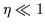In this limit, the obvious expansion parameter is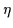. The unperturbed ground-state energy,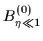, of an even-even system with particle number N=2n (n stands for the number of pairs) is that of Eq. (2), i.e.,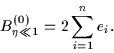(18)

The pairing Hamiltonian scatters the nucleonic pairs from hole states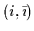to particle states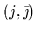, and the corresponding scattering matrix element is constant and equal to -G. The first order of perturbation theory gives the energy correction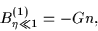(19)

while the second-order correction is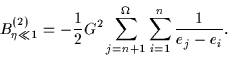(20)

In the case of an odd system with N=2n+1, the analogous expressions can be obtained by the following simple modifications. First, in the zero order, the (n+1)-th level is occupied (blocked) by one particle, and hence the single-particle energy en+1 should be added to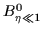. Moreover, since the pairing Hamiltonian does not couple orbitals occupied by one nucleon (the blocking effect), the orbital containing the odd particle must be excluded from the sum in Eq. (20), and the number of pairs in Eqs. (19) and (20) must become n=(N-1)/2.

Adding together the zero-, first-, and second-order contributions to the binding energy, one obtains the corresponding expressions for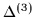: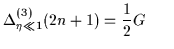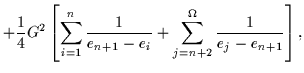(21)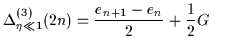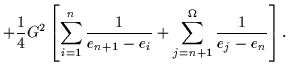(22)

Finally, for the single-particle energy-splitting filters (7) and (8), one obtains: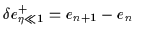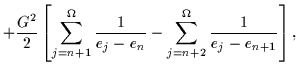(23)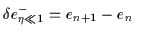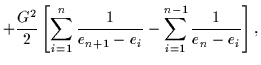(24)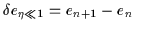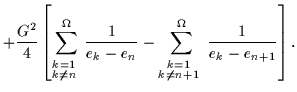(25)

It is clear that in the limit of small G (small compared to the typical single-particle energy spacing), the energy-spacing filters correctly extract the single-particle spectrum from the total binding energies.Next: Strong-pairing limit, Up: Limiting cases Previous: Limiting cases
Jacek Dobaczewski
2000-03-09